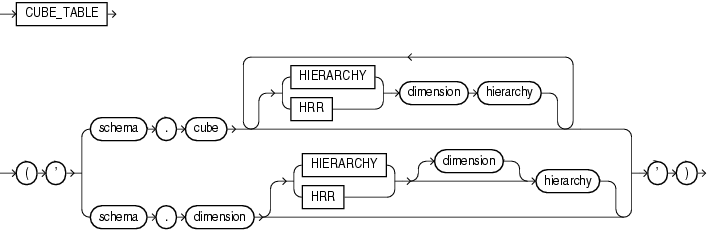## CUBE_TABLE

SyntaxDescription of the illustration cube_table.eps

Purpose

`CUBE_TABLE` extracts data from a cube or dimension and returns it in the two-dimensional format of a relational table, which can be used by SQL-based applications.

The function takes a single `VARCHAR2` argument. The optional hierarchy clause enables you to specify a dimension hierarchy. A cube can have multiple hierarchy clauses, one for each dimension.

You can generate these different types of tables:

• A cube table contains a key column for each dimension and a column for each measure and calculated measure in the cube. To create a cube table, you can specify the cube with or without a cube hierarchy clause. For a dimension with multiple hierarchies, this clause limits the return values to the dimension members and levels in the specified hierarchy. Without a hierarchy clause, all dimension members and all levels are included.

• A dimension table contains a key column, and a column for each level and each attribute. It also contains a `MEMBER_TYPE` column, which identifies each member with one of the following codes:

• `L` - Loaded from a table, view, or synonym

• `A` - Loaded member and the single root of all hierarchies in the dimension, that is, the "all" aggregate member

• `C` - Calculated member

All dimension members and all levels are included in the table. To create a dimension table, specify the dimension without a dimension hierarchy clause.

• A hierarchy table contains all the columns of a dimension table plus a column for the parent member and a column for each source level. It also contains a `MEMBER_TYPE` column, as described for dimension tables. Any dimension members and levels that are not part of the named hierarchy are excluded from the table. To create a hierarchy table, specify the dimension with a dimension hierarchy clause.

`CUBE_TABLE` is a table function and is always used in the context of a `SELECT` statement with this syntax:

`SELECT ... FROM TABLE(CUBE_TABLE('arg'));`

• Oracle OLAP User’s Guide for information about dimensional objects and about the tables generated by `CUBE_TABLE`.

• Appendix C in Oracle Database Globalization Support Guide for the collation derivation rules, which define the collation assigned to each character data type column in the table generated by `CUBE_TABLE`

Examples

The following examples require Oracle Database with the OLAP option and the `GLOBAL` sample schema. Refer to Oracle OLAP User’s Guide for information on downloading and installing the `GLOBAL` sample schema.

The following `SELECT` statement generates a dimension table of `CHANNEL` in the `GLOBAL` schema.

```SELECT dim_key, level_name, long_description, channel_total_id tot_id,
channel_channel_id chan_id, channel_long_description chan_desc,
total_long_description tot_desc
FROM TABLE(CUBE_TABLE('global.channel'));

DIM_KEY      LEVEL_NAME LONG_DESCRIPTION TOT_ID CHAN_ID CHAN_DESC    TOT_DESC
-----------  ---------- ---------------- ------ ------- ------------ -------------
CHANNEL_CAT  CHANNEL    Catalog          TOTAL  CAT     Catalog      Total Channel
CHANNEL_DIR  CHANNEL    Direct Sales     TOTAL  DIR     Direct Sales Total Channel
CHANNEL_INT  CHANNEL    Internet         TOTAL  INT     Internet     Total Channel
TOTAL_TOTAL  TOTAL      Total Channel    TOTAL                       Total Channel
```

The next statement generates a cube table of `UNITS_CUBE`. It restricts the table to the `MARKET` and `CALENDAR` hierarchies.

```SELECT sales, units, cost, time, customer, product, channel
FROM TABLE(CUBE_TABLE('global.units_cube HIERARCHY customer market HIERARCHY time calendar'))
WHERE rownum < 20;

SALES      UNITS       COST TIME                       CUSTOMER       PRODUCT     CHANNEL
---------- ---------- ---------- -------------------------- -------------- ----------- -----------
24538587.9      61109 22840853.7 CALENDAR_QUARTER_CY1998.Q1 TOTAL_TOTAL    TOTAL_TOTAL TOTAL_TOTAL
24993273.3      61320   23147171 CALENDAR_QUARTER_CY1998.Q2 TOTAL_TOTAL    TOTAL_TOTAL TOTAL_TOTAL
25080541.4      65265 23242535.4 CALENDAR_QUARTER_CY1998.Q3 TOTAL_TOTAL    TOTAL_TOTAL TOTAL_TOTAL
26258474      66122 24391020.6 CALENDAR_QUARTER_CY1998.Q4 TOTAL_TOTAL    TOTAL_TOTAL TOTAL_TOTAL
32785170      77589 30607218.1 CALENDAR_QUARTER_CY1999.Q1 TOTAL_TOTAL    TOTAL_TOTAL TOTAL_TOTAL
. . .```